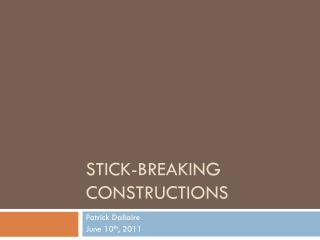Download PresentationStick-Breaking Constructions

# Stick-Breaking Constructions

Download Presentation## Stick-Breaking Constructions

- - - - - - - - - - - - - - - - - - - - - - - - - - - E N D - - - - - - - - - - - - - - - - - - - - - - - - - - -
##### Presentation Transcript

1. Stick-Breaking Constructions Patrick Dallaire June 10th, 2011

2. Outline • Introduction of the Stick-Breaking process

3. Outline • Introduction of the Stick-Breaking process • Presentation of fundamental representation

4. Outline • Introduction of the Stick-Breaking process • Presentation of fundamental representation • The Dirichlet process • The Pitman-Yor process • The Indian buffet process

5. Outline • Introduction of the Stick-Breaking process • Presentation of fundamental representation • The Dirichlet process • The Pitman-Yor process • The Indian buffet process • Definition of the Beta process

6. Outline • Introduction of the Stick-Breaking process • Presentation of fundamental representation • The Dirichlet process • The Pitman-Yor process • The Indian buffet process • Definition of the Beta process • A Stick-Breaking construction of Beta process

7. Outline • Introduction of the Stick-Breaking process • Presentation of fundamental representation • The Dirichlet process • The Pitman-Yor process • The Indian buffet process • Definition of the Beta process • A Stick-Breaking construction of Beta process • Conclusion and current work

8. The Stick-Breaking process

9. The Stick-Breaking process • Assume a stick of unit length

10. The Stick-Breaking process • Assume a stick of unit length

11. The Stick-Breaking process • Assume a stick of unit length • At each iteration, a part of the remaining stick is broken by sampling the proportion to cut

12. The Stick-Breaking process • Assume a stick of unit length • At each iteration, a part of the remaining stick is broken by sampling the proportion to cut

13. The Stick-Breaking process • Assume a stick of unit length • At each iteration, a part of the remaining stick is broken by sampling the proportion to cut

14. The Stick-Breaking process • Assume a stick of unit length • At each iteration, a part of the remaining stick is broken by sampling the proportion to cut

15. The Stick-Breaking process • Assume a stick of unit length • At each iteration, a part of the remaining stick is broken by sampling the proportion to cut

16. The Stick-Breaking process • Assume a stick of unit length • At each iteration, a part of the remaining stick is broken by sampling the proportion to cut

17. The Stick-Breaking process • Assume a stick of unit length • At each iteration, a part of the remaining stick is broken by sampling the proportion to cut

18. The Stick-Breaking process • Assume a stick of unit length • At each iteration, a part of the remaining stick is broken by sampling the proportion to cut

19. The Stick-Breaking process • Assume a stick of unit length • At each iteration, a part of the remaining stick is broken by sampling the proportion to cut

20. The Stick-Breaking process • Assume a stick of unit length • At each iteration, a part of the remaining stick is broken by sampling the proportion to cut

21. The Stick-Breaking process • Assume a stick of unit length • At each iteration, a part of the remaining stick is broken by sampling the proportion to cut

22. The Stick-Breaking process • Assume a stick of unit length • At each iteration, a part of the remaining stick is broken by sampling the proportion to cut • How should we sample these proportions?

23. Beta random proportions • Let be the proportion to cut at iteration

24. Beta random proportions • Let be the proportion to cut at iteration • The remaining length can be expressed as

25. Beta random proportions • Let be the proportion to cut at iteration • The remaining length can be expressed as • Thus, the broken part is defined by

26. Beta random proportions • Let be the proportion to cut at iteration • The remaining length can be expressed as • Thus, the broken part is defined by • We first consider the case where

27. Beta distribution • The Beta distribution is a density function on • Parameters and control its shape

28. The Dirichlet process

29. The Dirichlet process • Dirichlet processes are often used to produce infinite mixture models

30. The Dirichlet process • Dirichlet processes are often used to produce infinite mixture models • Each observation belongs to one of the infinitely many components

31. The Dirichlet process • Dirichlet processes are often used to produce infinite mixture models • Each observation belongs to one of the infinitely many components • The model ensures that only a finite number of components have appreciable weight

32. The Dirichlet process • A Dirichlet process, , can be constructed according to a Stick-Breaking process • Where is the base distribution and is a unit mass at

33. Construction demo

34. Construction demo

35. Construction demo

36. Construction demo

37. Construction demo

38. Construction demo

39. Construction demo

40. Construction demo

41. Construction demo

42. Construction demo

43. Construction demo

44. Construction demo

45. Construction demo

46. Construction demo

47. Construction demo

48. Construction demo

49. The Pitman-Yor process

50. The Pitman-Yor process • A Pitman-Yor process, , can be constructed according to a Stick-Breaking process • Where and